# Short Notes: Motion & Time Notes | Study Science Class 7 - Class 7

## Class 7: Short Notes: Motion & Time Notes | Study Science Class 7 - Class 7

The document Short Notes: Motion & Time Notes | Study Science Class 7 - Class 7 is a part of the Class 7 Course Science Class 7.
All you need of Class 7 at this link: Class 7

Time and Motion

Motion: When an object changes its position with elapse of time in respect to a stationary object, such as a building, electric pole, etc., the object is said to be in motion.

Slow and Fast Motion: If an object ‘A’ covers a distance in less time and another object covers the same distance in more time, the object A is called a faster moving object and object B is called a slower moving object. In other words, object A has faster motion and object B has slower motion.

Rectilinear Motion: Motion along a straight line is known as RECTILINEAR MOTION OR MOTION ALONG A STRAIGHT LINE. For example: Motion of a car along a straight line

Curvilinear Motion: Motion along a curve line is known as CURVILINEAR MOTION. For example: Motion of car or any moving object along a curve line

Circular Motion: Motion along a circle is known as CIRCULAR MOTION. For example: Motion of cyclist along a circular park.Speed: Distance covered by an object in unit time is called speed.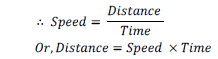Speed may be divided into three types: Uniform speed, Non-Uniform Speed and Average Speed

Uniform Speed: If an object covers a fixed distance in each unit of time the motion is known as UNIFORM MOTION or UNIFORM SPEED.

Non-uniform Speed: If an object does not cover a fixed distance in each unit of time the motion is known as NON-UNIFORM MOTION or NON-UNIFORM SPEED.

Average Speed: Total distance covered divided by total time taken is known as AVERAGE SPEED.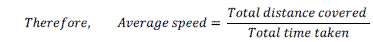Review Questions:

Question: 1 – A car covers a distance of 100 km in 5 hour, calculate its speed.

Solution:

Given, Total distance = 100 km

Total time taken = 5 hour

We know that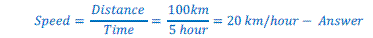Question: 2 – A car covers 20 km in the first hour and cover 30 km in the last 4 hours. Find its average speed.

Solution:

Given,

Total distance covered = 20 km + 30 km = 50 km

Total time taken = 1 hour + 4 hour = 5 hour

We know that,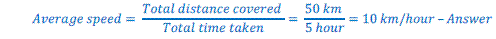Periodic Motion or Oscillatory Motion: When an object repeats its motion after every fixed interval of time, the motion of the object is called PERIODIC MOTION OR OSCILLATORY MOTION.

In many wall clocks pendulum is used even today. A device having a string suspended with a fixed point with a bob at bottom is called pendulum.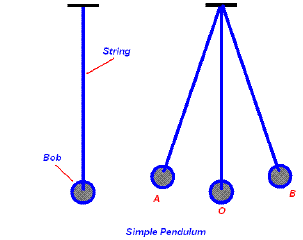The motion of pendulum starting from one extreme end to another extreme end and back to the first extreme end is called one oscillation. Similarly, the motion of a simple pendulum from its mean position to extreme left and extreme right and back to the mean position is called one oscillation.

Time Period: Time taken to complete one oscillation by the pendulum is called time period.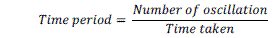Review Question:

Question: 1 – A pendulum takes 20 second to complete 10 oscillations, calculate its time period.

Solution:

Given, Number of oscillations = 10

Time taken = 20 second

We know that,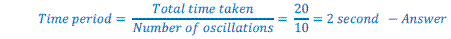Unit of Time: Time is measured in second. Thus, unit of time is second. Second is denoted by ‘s’.

60 second = 1 minute

60 minute = 1 hour

24 hour = 1 day

7 day = 1 week

365 days = 1 year

Unit of speed: Unit of speed is meter/second (m/s), or metre/minute (m/min) or kilometer/hour (km/h).

1000 metre (m) = 1 kilometer (km)

Speedometer: A device used to measure the speed of a vehicle is called SPEEDOMETER.

Odometer: A device used to measure the distance covered by a vehicle.

Distance Time Graph: When distance covered by an object and time taken to cover the distance is represented on a graph, the graph is called distance time graph.

If a vehicle cover a distance of 5 km every hour and travels for 5 hours, the time distance time graph for the given vehicle can be plotted as follows using the table given below: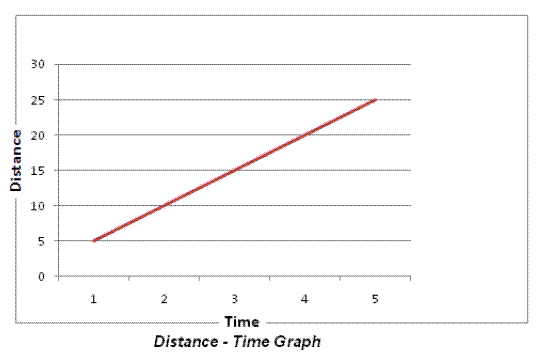Distance time graph of a moving vehicle with a constant speed is a straight slanting line.

Speed-time graph for the same vehicle will be a straight line parallel to x-axis.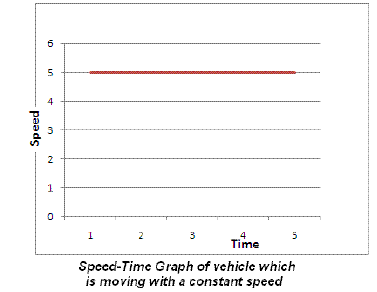The document Short Notes: Motion & Time Notes | Study Science Class 7 - Class 7 is a part of the Class 7 Course Science Class 7.
All you need of Class 7 at this link: Class 7Use Code STAYHOME200 and get INR 200 additional OFF

## Science Class 7

161 videos|216 docs|54 tests

Track your progress, build streaks, highlight & save important lessons and more!

,

,

,

,

,

,

,

,

,

,

,

,

,

,

,

,

,

,

,

,

,

;